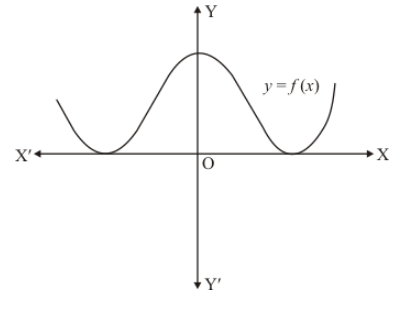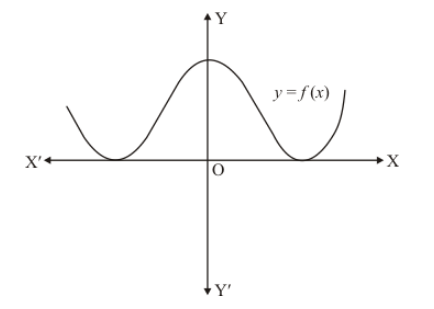# The graph of a polynomial f(x) is as shown in Fig. 2.21.`
Question:

The graph of a polynomial f(x) is as shown in Fig. 2.21. Write the number of real zeros of f(x).Solution:

The graph of a polynomial $f(x)$ touches $x$-axis at two pointsWe know that if a curve touches the $x$-axis at two points then it has two common zeros of $f(x)$.

Hence the number of zeros of $f(x)$, in this case is 2 .